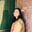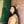Trusted answers to developer questions
Trusted Answers to Developer Questions

Related Tags

machine learning

# What is Stochastic gradient descent?Umme Ammara

Grokking Modern System Design Interview for Engineers & Managers

Ace your System Design Interview and take your career to the next level. Learn to handle the design of applications like Netflix, Quora, Facebook, Uber, and many more in a 45-min interview. Learn the RESHADED framework for architecting web-scale applications by determining requirements, constraints, and assumptions before diving into a step-by-step design process.

## Overview

In machine learning, we use gradient descent as an optimization technique to find the optimal model parameters that would result in the minimal cost value.

### Types of gradient descent

Depending on the implementation, there are three types of gradient descent algorithms.

1. Batch gradient descent

2. Stochastic gradient descent

3. Mini-Batch gradient descent

In this shot, we’ll be focusing on Stochastic gradient descent.

### Stochastic gradient descent

We use the entire dataset to calculate the gradient for every iteration in a standard gradient approach. The downside of this approach is recognized when the dataset size increases considerably. For each iteration, all the dataset samples will be used until a minimum is found, making the algorithm inefficient and resource-intensive.

Therefore, a better approach is to use the Stochastic Gradient Descent (SGD), in which a few dataset items are sampled and used for each iteration. This sample is collected randomly after shuffling the dataset.

Due to the randomization involved in SGD, it takes more iterations to reach the minima, and the path/s taken to get that minima are noisier.

This is illustrated in the image below.

Path taken to reach the minima

### Code

Let’s look at an example pseudocode implementation of SGD in Python.

def SGD(theta0, learning_rate, no_iterations):    i = 0    theta = theta0    for i in range(i+1, no_iterations+1):        cost, gradient = predict(theta)        theta = theta - (learning_rate * gradient)

### Explanation

In the code above, theta0 is the initial point from where the SGD is started, learning_rate is the learning rate of the algorithm, and no_iterations represents the total number of iterations for which the SGD process will be run.

We initialize a function that takes in three parameters. We’re assuming a predict function has been implemented that returns the cost and the gradient we’ll optimize. Once the iterations are exhausted, we produce the output as theta.

RELATED TAGS

machine learning

CONTRIBUTORUmme Ammara